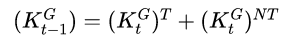# Timing of capital in two sector economy

Hello all,

I am building a two-sectoral model, and I have a question about the timing of sectoral-capital variables: In my model, T and N are sectors, hence kT is capital in sector T and kN is capital in sector N. Then, I assume that the economy’s aggregate capital is simply: k = kT + kN.

However, I assume only one law of motion for aggregate capital such that: k = (1-delta)*k(-1) + i - written in Dynare language. It is clear to me that k is a predetermined variable and should be treated as such in the code, however I am not very sure how kT and kN should be treated. As predetermined, or as normal ones?

Thanks a lot!

Kyriacos

In this case, only the total capital stock k is predetermined. Hence, you have to use a law of motion for capital

``k = (1-delta)*k(-1) + i``

The total capital stock in the economy k(-1), which was decided by investment in the period before (hence the (-1) timing), can be freely divided between the individual sectors. Hence, kT and kN are determined at time t and not predetermined (normal variables). The corresponding resource constraint thus reads

``` k(-1) = kT + kN```

1 Like

Dear all,

I have a similar model; two country two sector model with capital. I assume that investment can only be done by using the output of traded sector but capital can be used in the production of both sectors. Even though I changed the timing of the resource constraint as you suggested, there are some issues with the results. The model solves however the results dont make any sense.

I would really appreciate any help regarding my model.

Best,
Ayse

You need to be more specific what the problem is.

Hi,
I am working on Smets and Wouters model and have introduced informal sector. I have achieved the steady state already. But the Blanchard-Kahn condition is not satisfied.
Could anyone help me out.thank you.

Have you checked whether the problem outlined here affects your model?

Hi,
Yes I do have capital in my model too but it’s determined by the utilisation rate in both sectors. So I have…
k_t=u_t*k_bar_t-1 and
k_bar_t is determined by the standard capital utilisation equation.
Does the timing condition also affect it?

You need to sort out how the timing works in this case for both sectors.

Hi,
Thank you very much. Its working perfectly.

Thanks very much professor. Does that mean that I have to change the timing of my kT and kN variables in all my code and not only in the constraint:
k(-1) = kT + kN

I mean should I change my timing also in the production function for good T changing:
yT=theta*(kT(-1))^(alpha)(Lt)^(1-alpha)
to
yT=theta
(kT^alpha)*Lt^(1-alpha)

and well this logic for every equation where I have a kT(-1) and kN(-1)

That depends on the assumptions in the paper, but typically, yes.

Hi Prof Pfeifer, I have a related question concerning the discussion here. In my two sector model, firms use both private and government capital.

Concerning private capital, I have these equations in my mod file as discussed here:However, regarding government capital, including the equation below makes the number of equilibrium equations in the mod file one larger than the number of endo variables.To get around this problem, this has been my solution so far:
Given the above equation, I can rewrite it as follows using some weights:So that I have the following production functions in my mod file:In this case, I am able to exclude the resource constraint for government capital from the equilibrium equations in the mod file, allowing for the number equations to match the number of endo variables.

The model works as expected, but is this procedure an good alternative to specifying a resource constraints for government capital? That is,.

I am not sure I understand the setup. Given that government capital is taken as given by private agents, there is nothing here that would determine the split between the two sectors. So unless there are conditions that determine this choice (as you do with your weights), this cannot work.

Hi Prof. Pfeifer, many thanks for the reply. I am just experimenting with this setup. Here, the government does the split and the weights are calibrated. After log-linearizing the production functions, the weights disappear though, so maybe they are irrelevant in a linear model.